## Polar Circle

Given a Triangle, the polar circle has center at the Orthocenter. Call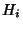the Feet of the Altitude. Then the Radius is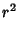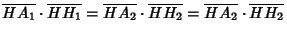(1)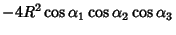(2)(3)

whereis the Circumradius,the Vertex angles, and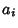the corresponding side lengths.

A Triangle is self-conjugate with respect to its polar circle. Also, the Radical Axis of any two polar circles is the Altitude from the third Vertex. Any two polar circles of an Orthocentric System are orthogonal. The polar circles of the triangles of a Complete Quadrilateral constitute a Coaxal System conjugate to that of the circles on the diagonals.